﻿ Feynman propagators of scalar, Dirac, photon fields

# Propagators of scalar, Dirac and photon fields.

## Propagator of Klein-Gordon field.

(Fig.1) Internal lines = propagator.Propagator is a main tool in calculating various values in quantum electrodynamics.
QED propagators were introduced by changing classical Lorentz force into relativistic interaction.
This means if special relativity is wrong, these interactions using propagators are wrong.

In this section, we explain feynman propagators in various fields ( scalar, Dirac, and electromagnatic fields.)
To understand propagators, we need to understand the basic quantum field theory first.

### [ Propagator in scalar ( Klein-Gordon ) field . ]

The plain wave solution of real Klein-Gordon field is
(Eq.1)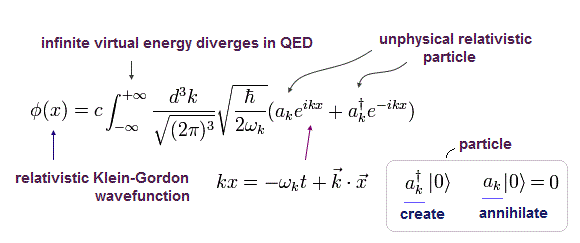Caution: here we use (-1, 1, 1, 1) version metric tensor.
If you want to use (1, -1, -1, -1) version, change notation like ( kx → -kx, k2 → -k2 ).
The meanings of them are completely the same. (Only notations are different.)

Propagator ( Green function ) of the scalar field is expressed as
(Eq.2)where θ(t-t') means Heaviside step function of
(Eq.3)This is used for time-ordered.
In the step function (= θ ) of Eq.3, when (t-t') is positive, θ becomes "1", and when t-t' is negative, θ becomes zero.

So Eq.2 means that the scalar particles are created first in the vacuum and later annihilated.
( Eq.1 includes "creation" ( a ) and "annihilation" ( a ) operators. )
Of course, as shown in Eq.1, propagator contains infinite particles of infinite kinds of momentums ( ħ k ).
( So the propagator itself is NOT a real thing. But QED uses this concept to get values. )

Using complex integral, step function can be expressed as
(Eq.4)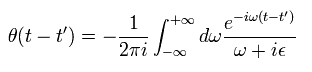and
(Eq.5)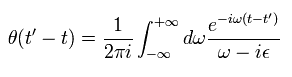where we make the exponential function parts the same.

--------------------------------------------------

### [ Proof of Eq.4 and Eq.5. ]

First, we prove Eq.4.
If you want to skip this proof, please proceed to the next section.
To prove Eq.4, we have to use Cauchy's residue theorem.

In the residue theorem, only the coefficient of 1/(z-a) is left by the complex integration.
(Eq.6)and
(Eq.7)where integral is counterclockwise closed contour.
And when the closed contour doesn't contain "pole", Eq.7 becomes zero.

(Eq.4)In Eq.4, when t-t' is positive ( t-t' > 0 ), the exponential parts of Eq.4 becomes zero in the region of ω = -i∞, as follows,
(Eq.8)So we can treat the integration from -∞ to +∞ along the real line as the complex integration of Fig.2.

(Fig.2) Step function ( complex integration ) of Eq.4. ( t-t' > 0 )As shown in Fig.2, "pole" exists in the minus imaginary area, so it doesn't become zero.
Expanding Eq.4, the coefficient c-1 becomes " 1 ", as follows,
(Eq.9)Fig.2 is clockwise closed contour, so the result becomes opposite ( 1 → -1 ).
(Eq.10)As a result, when t-t' > 0, the step function θ becomes "1",
(Eq.11)When t-t' is negative ( t-t' < 0 ), the exponential part of Eq.4 becomes zero, as ω → i∞,
(Eq.12)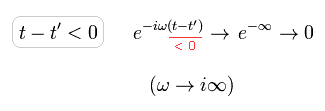So the integration of Eq.4 is equal to the counterclockwise closed contour in the complex field,
(Fig.3)As shown in Fig.3, this closed contour doesn't contain a pole.
So the step function θ becomes zero, when t-t' is negative.

Next we think about the step function of Eq.5.
(Eq.5)(Fig.4) Step function of Eq.5.In Eq.5, "pole" exists in the plus imaginary area, as shown in Fig.4.
In the same way as Eq.4, we can get the results of
(Eq.13)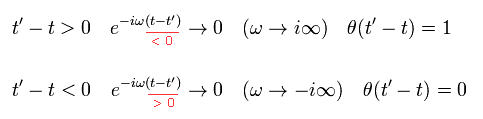---------------------------------------------

### [ Propagator. ]

(Eq.1) Klein-Gordon wavefunction.The creation and annihilation operators of Eq.1 satisfy the following commutation relation.
(Eq.14)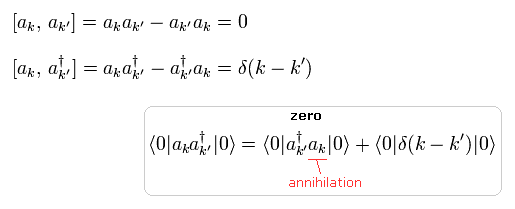Using two different wavefunctions of Eq.1, we calculate
(Eq.15)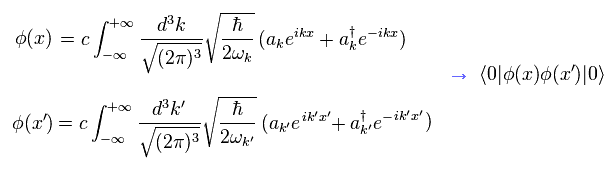Due to the relation between the vacuum of both sides and annihilation operators, only aa term is left
(Eq.16)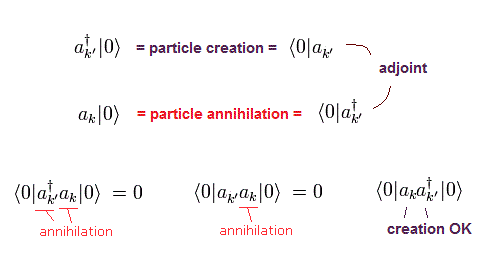Using the commutation relation of Eq.14, the result of Eq.15 becomes
(Eq.17)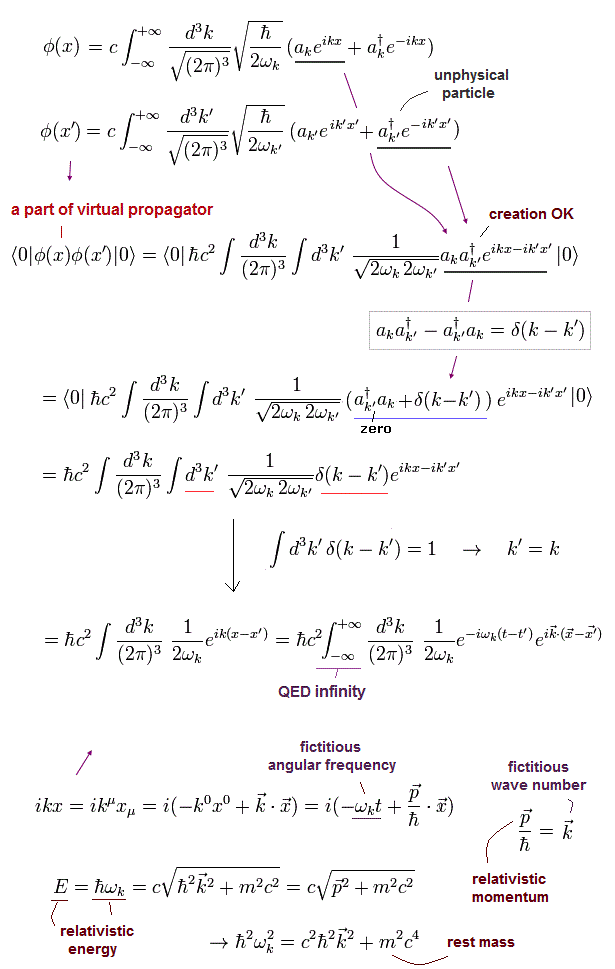In the same way, exchanging x and x' of Eq.17,
(Eq.18)In Eq.18, ωk is an even function of ki, as follows,
(Eq.19)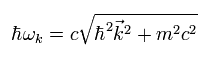So if ki values are determined, ωk is also determined, and it's always positive.
Changing the sign of 1-3 component ki ( k → -k ) in Eq.18, the exponential function of momentum ( eikx ) parts become the same as Eq.17, as follows,
(Eq.20)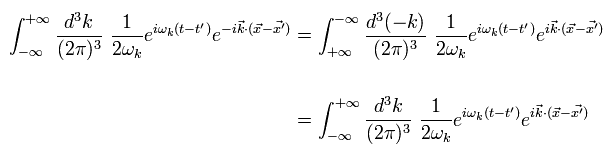Using Eq.4 and Eq.17, the first term of Eq.2 is
(Eq.21)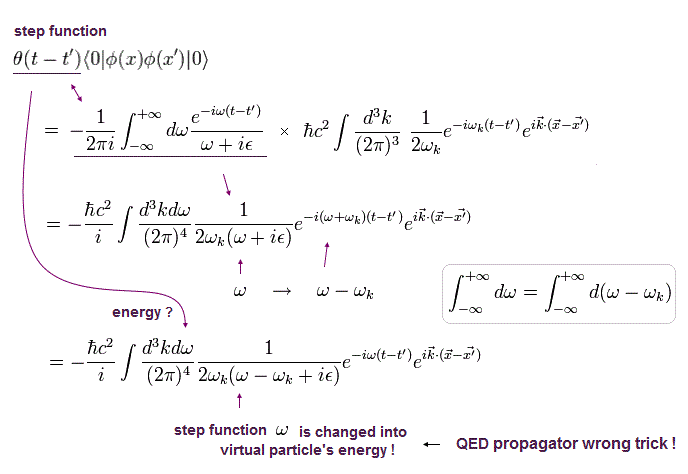From Eq.5 and Eq.18 (Eq.20), the second term of Eq.2 is
(Eq.22)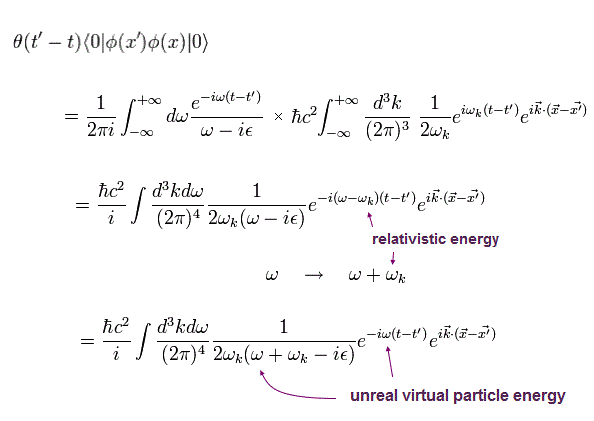Changing ω to k0, as follows,
(Eq.23)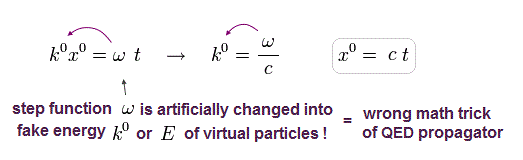As a result, the Klein-Gordon propagator of Eq.2 is the sum of Eq.21 and Eq.22, as follows,
(Eq.24)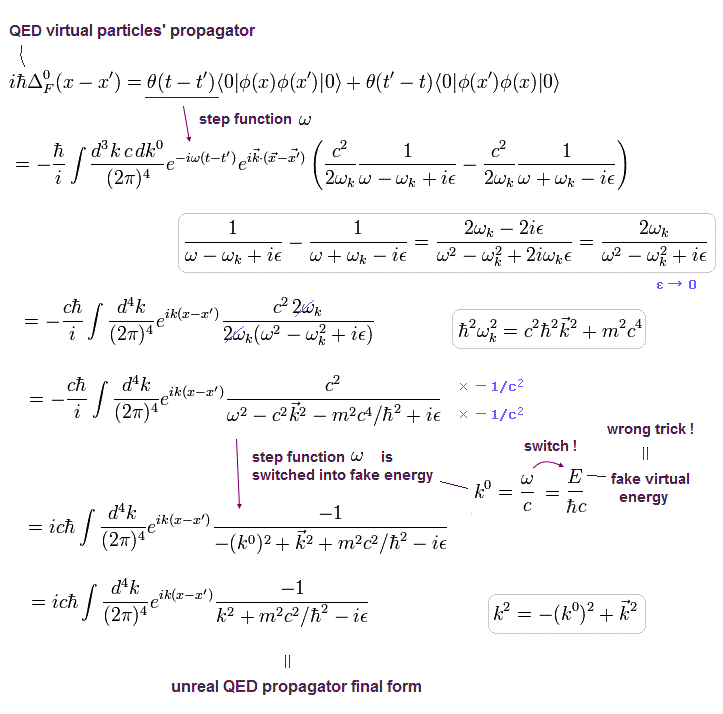This is what is called "propagator" (= Green's function ) of scalar field.
And the scalar propagator is a basis for other fields, so it is very important.

## Propagator of Dirac field.

In QED, Dirac propagator is mainly used. So next we explain Dirac field.
(Eq.25)And its conjugate transpose (× γ0) is
(Eq.26)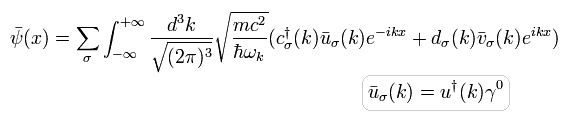And the particle ( c, c ) and antiparticle ( d, d ) satisfy the anticommutation relations of
(Eq.27)Other combinations become zero at the right hand sides.

Dirac propagator is expressed as
(Eq.28)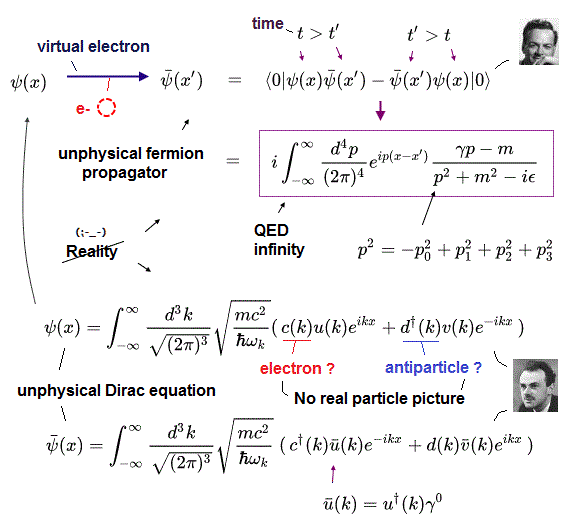Dirac field satisfies anticommutation relation, so the "minus" sign is added in the second term of Eq.28.

Considering annihilation by the vacuum at both ends, only "cc" is left.
(Eq.29)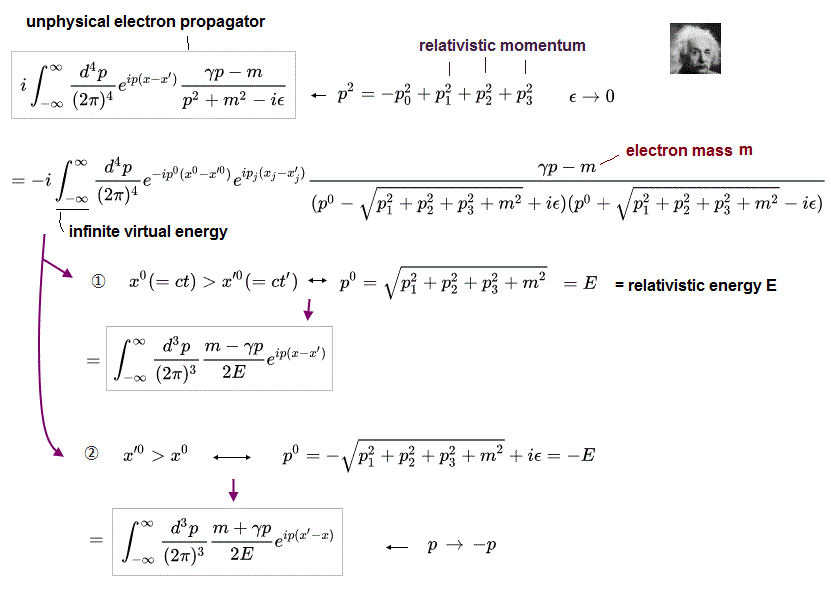From Eq.29, we get
(Eq.30)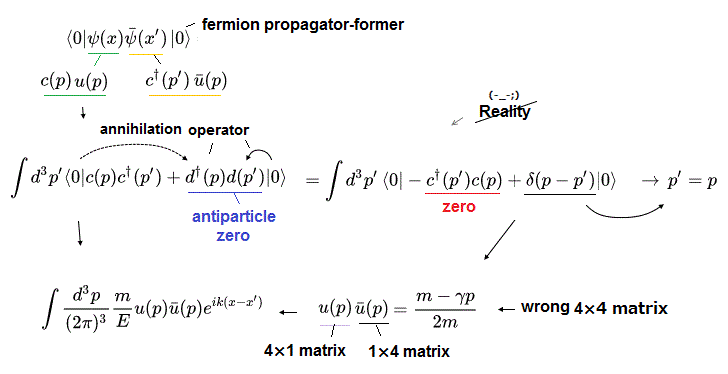where anticommutation of Eq.27 is used.

The important point is that matrix parts (= u ) of Eq.30 can not be numbers, as follows,
(Eq.31)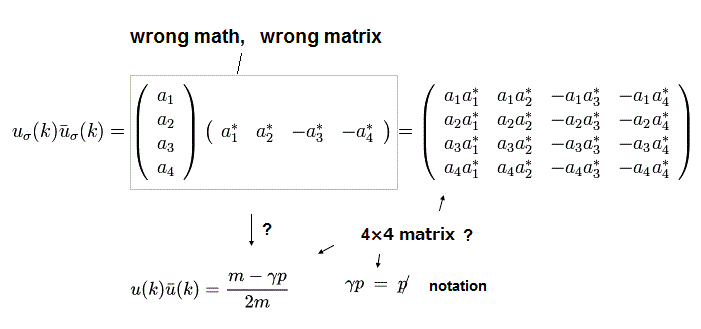To put it simply, the two matrices u only stand in line in Eq.31.

The state of Eq.31 can be expressed as ,
(Eq.32)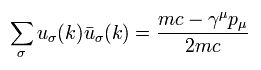If two matrices u of Eq.32 only stand in line (not united into number), when we multiply Eq.32 by another u(k) from right side,
(Eq.33)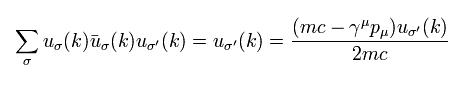where we use the relation of
(Eq.34)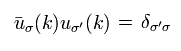Eq.33 is equal to
(Eq.35)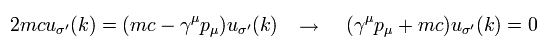Eq.35 is equal to Eq.5-12 of this page, which matrix u(k) needs to satisfy.
As a result, Eq.32 is proved.

In the same way, we can define
(Eq.36)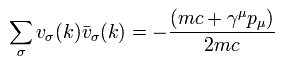Adding another v(k) from the right side of Eq.36, we get
(Eq.37)Using Eq.32, Eq.30 becomes
(Eq.38)In calculating the second term of Dirac propagator (Eq.28), " c c " term is annihilated.
(Eq.39)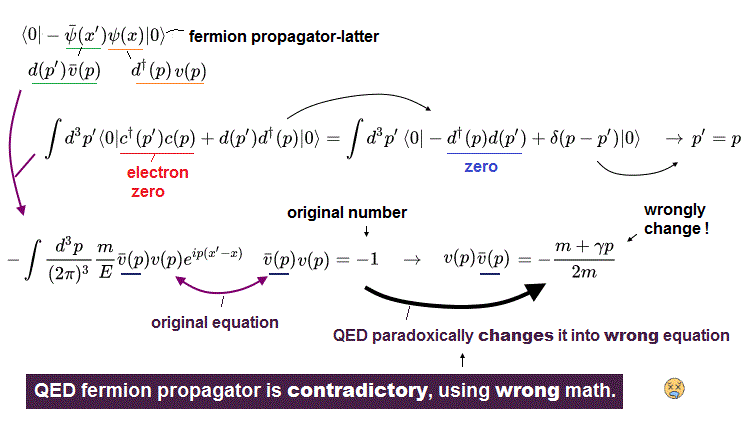where " d d " term is left.
(Eq.40)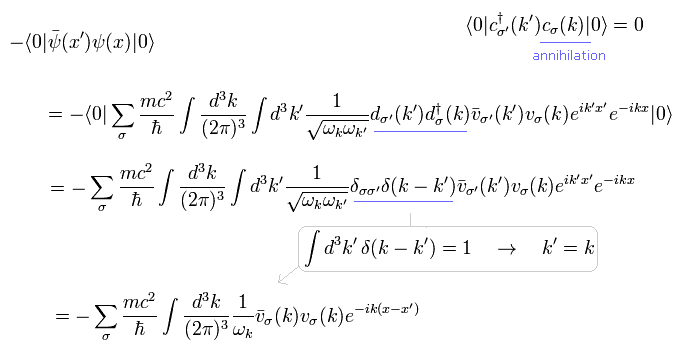As you notice, the two matrices part v(k) of Eq.40 is a row matrix × a column matrix.
So Eq.40 is completely different from Eq.30.
To use Dirac's propagator, we have to make them the same kind thing "artificially".

Using Eq.36, Eq.40 becomes
(Eq.41)Note that coefficient pμ of Eq.41 originally means Dirac's differential operators.
So if the exponential function changes (at the final form ), they also change as
(Eq.42)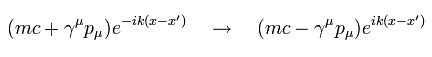So basically, Dirac propagator resembles Klein-Gordon propagator of Eq.24 except for their coefficients, as follows,

(Eq.43) Klein-Gordon propagator.(Eq.44) Dirac propagator.Comparing Eq.43 and Eq.44, Dirac propagator is
(Eq.45)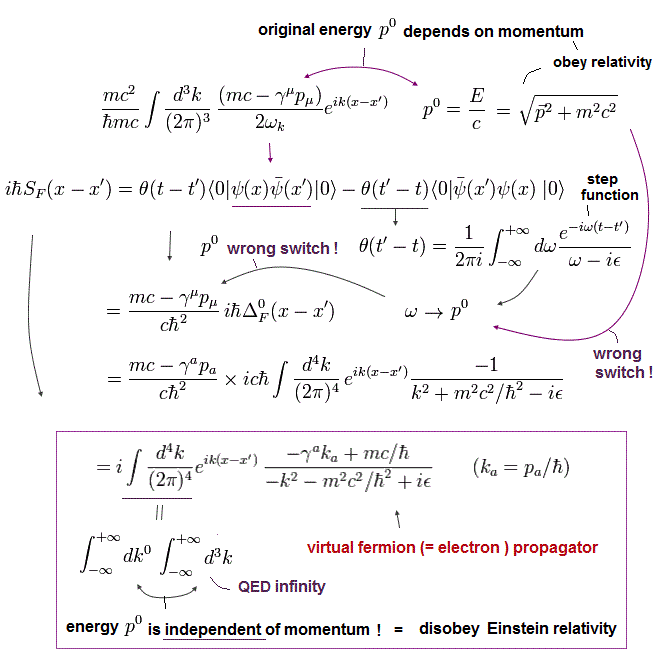Eq.45 is Dirac's propagator, which is often used in QED.

## Propagators of photons and strange " zero " component ?

### [ Zero component of photons and propagators. ]

As shown on this page, Lagrangian of the electromagnetic field in the vacuum can be expressed as
(Eq.46)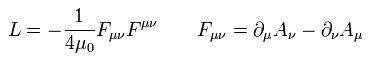As shown in Eq.46, if μ and ν are the same, Fμν becomes zero.
So the zero component of the canonical momentum is zero, as follows,
(Eq.47)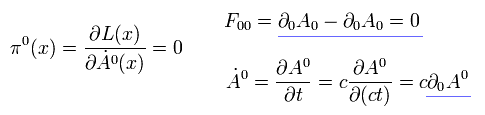But as is explained later, if the zero component of photon can not be defined, the propagator calculation becomes impossible.
So we have to produce the zero component canonical momentum "compulsorily".

We define Lagrangian which includes the zero component, as follows,
(Eq.48)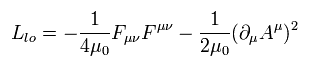This is called " Lagrangian of Feynman gauge ".
( Here we use usual SI unit, so magnetic permeability μ0 is added. )

The second term of Eq.48 vanishes if we consider Lorentz gauge condition of
(Eq.49)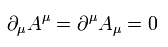So the cannonical momentum of the zero component is, ( x0 = ct )
(Eq.50)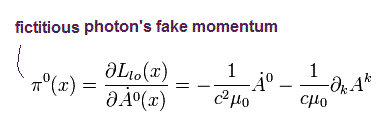where Lagrangian involved is
(Eq.51)And the canonical momentums of other components ( j = 1, 2, 3 ) are
(Eq.52)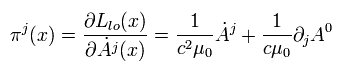where Lagrangian involved is
(Eq.53)μ and ν of Eq.48 contain all 0 - 3 components.
So the coefficient 1/4 (and 1/2) is cancelled out.

Like de Broglie's relation, we can define the commutation relations of
(Eq.54)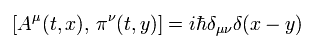If so,
(Eq.55)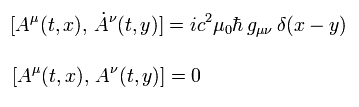where gμν appears.
Other combinations are all zero.

Using Eq.55, we can prove Eq.54, as follows,
(Eq.56)and
(Eq.57)Here (-1,1,1,1) version metric tensor g is used.

As shown on this page, the solution of Aμ (x), which satisfies Maxwell equation is
(Eq.58)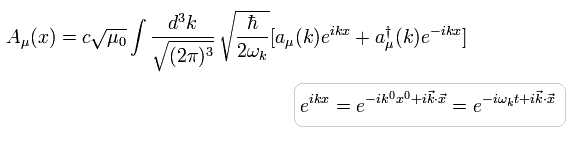The time derivative of Eq.58 is
(Eq.59)And to satisfy Eq.55, the following relations must be valid.
(Eq.60)Other combinations are all zero.

As shown in Eq.60, only the zero component of photon particles has "negative" energy ( = "negative" number ). ( g00 = -1 )
So this zero component photon is NOT a real thing.
Of course, if we consider Lorentz gauge condition of Eq.49, this zero component photon can be removed.
This strange property is caused by the minus sign of the second term in Eq.48.
( This minus sign is artificially introduced. )

In fact, if we don't define the minus sign of Eq.60, we can NOT calculate the g-factor.
In other words, to calculate g-factor, the strange relation of Eq.60 is introduced.
This is explained later.

Next we prove Eq.55 using the relations of Eq.60.
Substituting Eq.58 and Eq.59 into Eq.55,
(Eq.61)where
(Eq.62)where time t is the same.

From Eq.61 and Eq.62 we have
(Eq.63)Eq.55 is gotten.

### [ Photon propagator. ]

Eq.58 resembles Eq.1.
So propagators of photons are almost same as those of Klein-Gordon field of Eq.24, except for zero photon mass
So the photon propagators are
(Eq.64)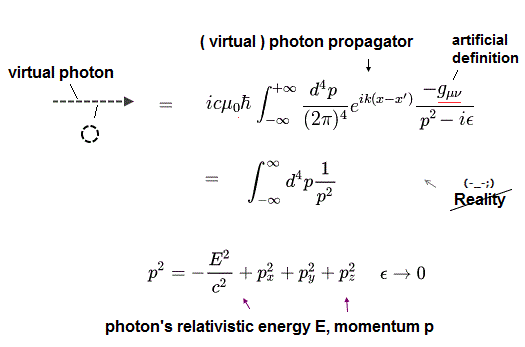So only in the zero component photon, the sign becomes opposite.
And photons are vectors, so propagators between photons of different components are zero, as shown in Eq.64.

### [ Coulomb gauge Hamiltonian. ]

Here we explain Hamiltonian of Coulomb gauge briefly.
When the scalar potential (= A0 ) is zero,
(Eq.65)Hamiltonian is
(Eq.66)Of course, in this webpage, we use Lorentz gauge, so we have to consider "minus" energy of the zero component photons in addition to this.2013/1/20 updated. Feel free to link to this site.